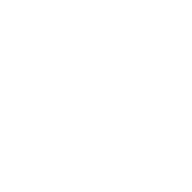# extrapolating the edges of a bounding box

·2 mins

I woke up thinking about a fragment of a map the other day, and it occurred to me that what I was picturing could probably be made using geometry generators in QGIS. I was picturing something like this:

So I thought I’d have a whack at making it.

This was my first foray into crafting a geometry generator, inspired by some blog posts I read recently from BOGIND and Alasdair Rae.

My approach was something like:

• browse the Geometry functions in the Expression Editor while pondering “how might I go about doing this…”
• arrive at the idea of doing some maths to the coordinates of the centroid to produce two points to draw one line with
• do that four times

After some fiddling around I arrived at the following geometry generator, which did the north-south extrapolation on the right edge of the bounding box:

``````# RIGHT

make_line(
make_point(
x(\$geometry) + bounds_width(\$geometry)/2,
y(\$geometry) + bounds_height(\$geometry)*18
),
make_point(
x(\$geometry) + bounds_width(\$geometry)/2,
y(\$geometry) + bounds_height(\$geometry)*18*-1
)
)
``````

Some adjustments to plusses and minuses and negatives did the other edges:

``````# LEFT

make_line(
make_point(
x(\$geometry) - bounds_width(\$geometry)/2,
y(\$geometry) + bounds_height(\$geometry)*18
),
make_point(
x(\$geometry) - bounds_width(\$geometry)/2,
y(\$geometry) + bounds_height(\$geometry)*18*-1
)
)
``````
``````# TOP

make_line(
make_point(
x(\$geometry) + bounds_width(\$geometry)*18,
y(\$geometry) + bounds_height(\$geometry)/2
),
make_point(
x(\$geometry) + bounds_width(\$geometry)*18*-1,
y(\$geometry) + bounds_height(\$geometry)/2
)
)
``````
``````# BOTTOM

make_line(
make_point(
x(\$geometry) + bounds_width(\$geometry)*18,
y(\$geometry) - bounds_height(\$geometry)/2
),
make_point(
x(\$geometry) + bounds_width(\$geometry)*18*-1,
y(\$geometry) - bounds_height(\$geometry)/2
)
)
``````

You may be wondering what the number `18` that repeats throughout is about. It is a multiplier that dictates how long the extrapolated lines are. I started off with a smaller number but increased it while playing around until the extrapolated lines extended out of the map area I was looking at, out of preference / what I was aiming for visually. (It didn’t occur to me there was a much simpler way of setting particular point coordinates to achieve this until I was recreating this in Leaftlet, doh! I’ll cover that later in a separate post…)